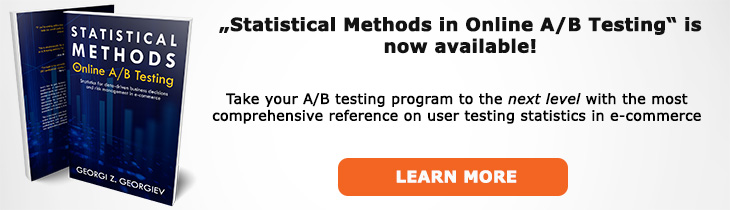# What does "Conversion Rate" mean?

Definition of Conversion Rate in the context of A/B testing (online controlled experiments).

## What is Conversion Rate?

Aliases: CR

Conversion rate is the proportion of conversions versus a base metric such as users, sessions, email subscribers, etc. with a generic formula being CR = C/N where C is the number of conversion events and N is the number of events during which a conversion could have occurred. It can be expressed as a proportion or percentage, for example a user-based conversion rate can be calculated from 10 conversions out of 100 users as 10/100 = 1/10 = 0.1 = 10%.

Different kinds of conversion rates are often used as a Primary KPI or a Secondary KPI since being a binomial metric makes them easy to work with due to their analytically known standard deviation.

If the revenue per conversion is equal or about equal across conversions, a conversion rate can be used as the metric closest to the business bottom-line. However, when revenue per conversion varies significantly the conversion rate becomes a proxy metric and one should consider measuring ARPU, AOV and other metrics instead despite the increased difficulty.

Binomial Metric

## Articles on Conversion Rate

Like this glossary entry? For an in-depth and comprehensive reading on A/B testing stats, check out the book "Statistical Methods in Online A/B Testing" by the author of this glossary, Georgi Georgiev.## Glossary Index by Letter

Select a letter to see all A/B testing terms starting with that letter or visit the Glossary homepage to see all.